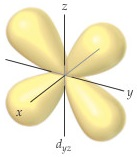# Problem: The accompanying drawing shows a contour plot for a dyz orbital. Consider the quantum numbers that could potentially correspond to this orbital.What is the value of the angular momentum quantum number, l?

###### FREE Expert Solution

Recall that the quantum numbers that define an electron are:

• the principal quantum number (n) → energy level in orbitals and its value could be any positive integer starting from 1

angular momentum quantum number (ℓ) → (l) has to be at least 1 less than n, range of values from 0 up to (n-1)

▪ Each ℓ value corresponds to a subshell/sublevel:

85% (229 ratings)###### Problem Details

The accompanying drawing shows a contour plot for a dyz orbital. Consider the quantum numbers that could potentially correspond to this orbital.What is the value of the angular momentum quantum number, l?